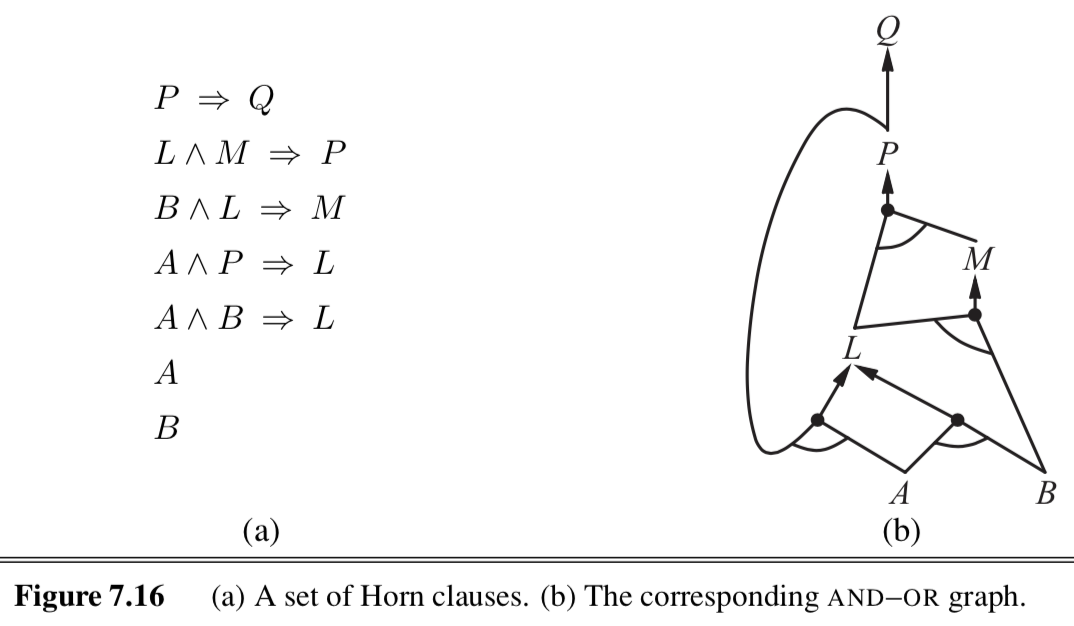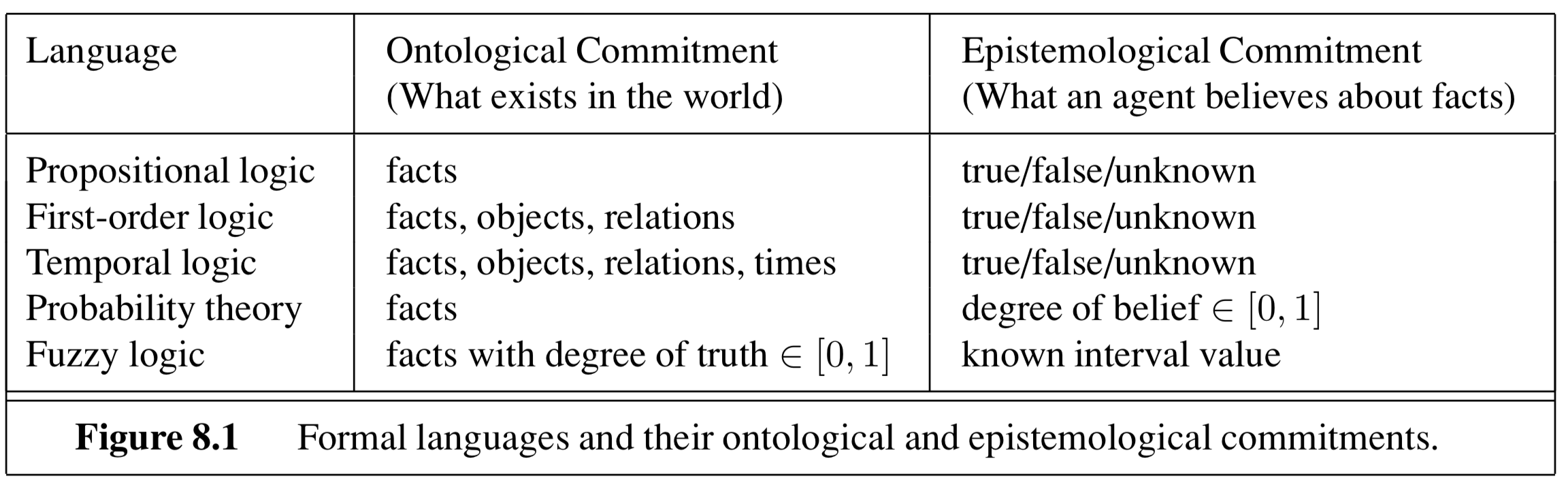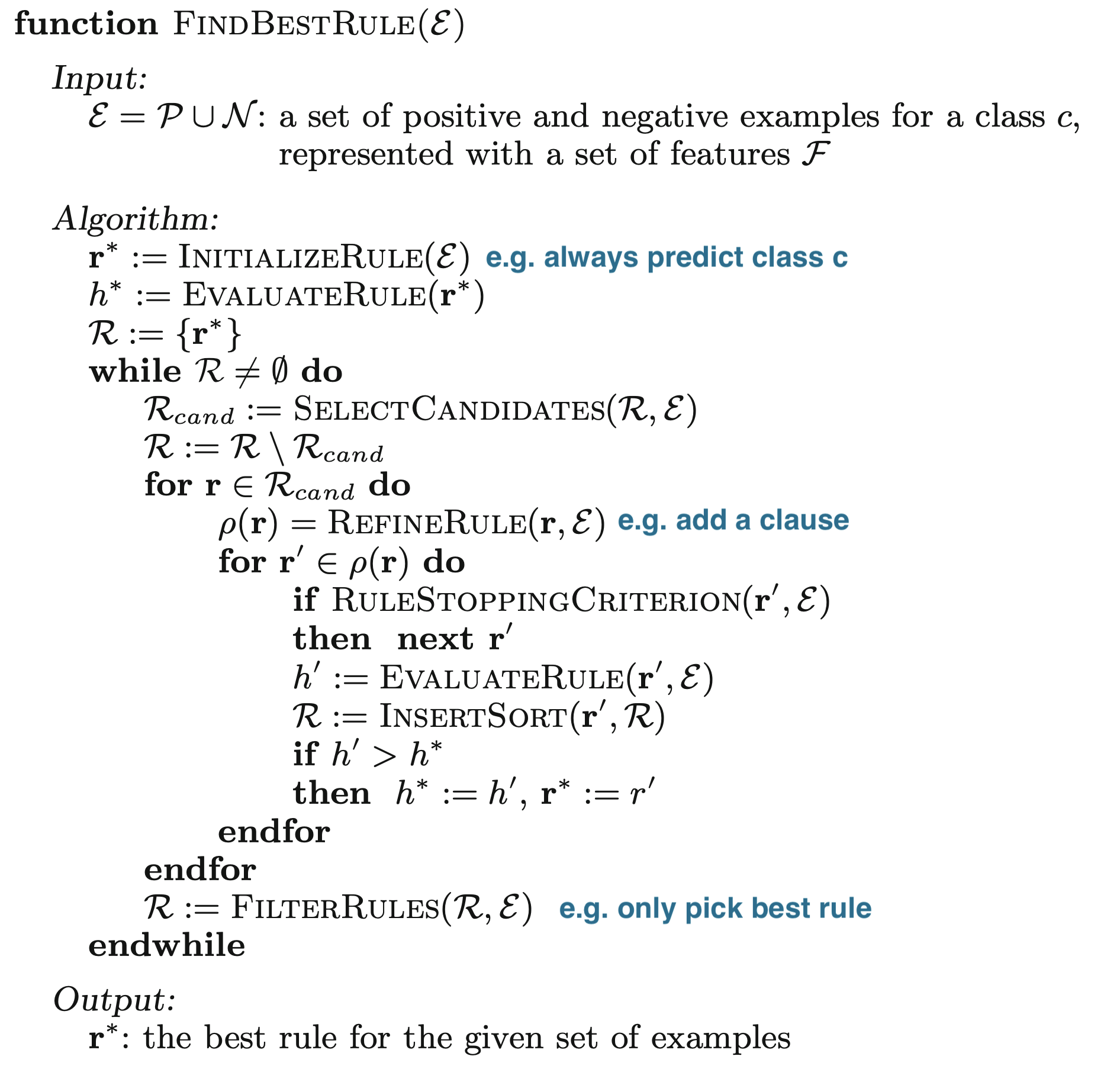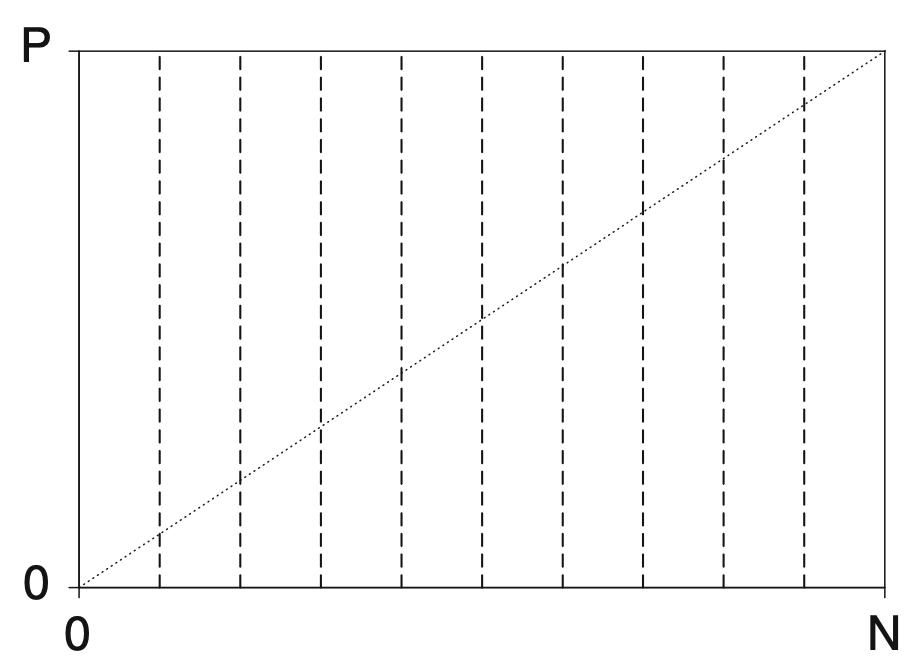Logic

Some notes on logic based on Berkeley's CS 188 course and "Artificial Intelligence" Russel & Norvig 3rd Edition + foundations of rule learning (furnkranz et al. 2014)

# logical agents - 7.1-7.7 (omitting 7.5.2)

• knowledge-based agents - intelligence is based on reasoning that operates on internal representations of knowledge
• deductive - general-to-specific
• inductive - specific-to-general
• 3 steps: given a percept, the agent
1. adds the percept to its knowledge base (KB)
2. asks the knowledge base for the best action
3. tells the knowledge base that it has taken that action
• 2 approaches
• declarative approach - tell sentences until agent knows how to operate
• know something, can verbalize it
• procedural approach - encodes desired behaviors as program code
• intuitively know how to do it (ex. riding a bike)
• ex. Wumpus World
• logical entailment between sentences
• $A \vDash B$ means B follows logically from A (A implies B)
• logical inference - showing entailment
• model checking - try everything to see if A $\implies$ B
• this is sound = truth-preserving
• complete - can derive any sentence that is entailed
• TT-ENTAILS
• recursively enumerate all sentences by assigning true, false to each variable
• check if these are valid within the KB
• if they are, they must also match the query (otherwise return false)
• grounding - connection between logic and real environment (usually sensors)
• theorem properties
• validity - tautalogy - true under all models
• satisfiable - true under some model
• ex. boolean-SAT
• monotonicity - set of implications can only increase as info is added to the knowledge base
• if $KB \implies A$ then $KB \land B \implies A$

## theorem proving

• resolution rule - resolves different rules with each other - leads to complete inference procedure
• CNF - conjunctive normal form - conjunction (and) of clauses (with ors)
• ex: $( \neg A \lor B) \land \neg C \land (D \lor E)$
• anything can be expressed as this
• horn clause - at most one positive
• definite clause - disjunction of literals with exactly one positive: ex. ($A \lor \neg B \lor \neg C$)
• goal clause - no positive: ex. ($\neg A \lor \neg B \lor \neg C$)
• benefits
• easy to understand
• forward-chaining / backward-chaining are applicable
• deciding entailment is linear in size (KB)
• forward/backward chaining: checks if q is entailed by KB of definite clauses

• data-driven
•• backward chaining works backwards from the query
• goal-driven
• keep going until get back to known facts
• checking satisfiability
• complete backtracking
• davis-putnam algorithm = DPLL - like TT-entails with 3 improvements
1. early termination
2. pure symbol heuristic - pure symbol appears with same sign in all clauses, can just set it to the proper value
3. unit clause heuristic - clause with just one literal or one literal not already assigned false
• other improvements (similar to search)
1. component analysis
2. variable and value ordering
3. intelligent backtracking
4. random restarts
5. clever indexing
• local search
• evaluation function can just count number of unsatisfied clauses (MIN-CONFLICTS algorithm for CSPs)
• WALKSAT - randomly chooses between flipping based on MIN-CONFLICTS and randomly
• runs forever if no soln
• underconstrained problems are easy to find solns
• satisfiability threshold conjecture - for random clauses, probability of satisfiability goes to 0 or 1 based on ratio of clauses to symbols
• hardest problems are at the threshold

## agents based on propositional logic

• fluents = state variables that change over time
• can index these by time
• effect axioms - specify the effect of an action at the next time step
• frame axioms - assert that all propositions remain the same under actions
• succesor-state axiom: $F^{t+1} \iff ActionCausesF^t \lor (F^t \land -ActionCausesNotF^t )$
• ex. $HaveArrow^{t+1} \iff (HaveArrow^t \land \neg Shoot^t)$
• makes things stay the same unles something changes
• state-estimation: keep track of belief state
• can just use 1-CNF (conjunctions of literals: ex. $WumpusAlive \land L_2 \land B$)
• 1-CNF includes all states that are in fact possible given the full percept history
• conservative approximation - contains belief state, but also extraneous stuff
• planning
• could use $A^*$ with entailment
• otherwise, could use SATPLAN
• SATPLAN - how to make plans for future actions that solve the goal by propositional inference
• basic idea
• make assertions
• transitions up to some max time $t_{final}$
• assert that goal is achieved at time $t_{final}$ (ex. havegold)
• present this to a SAT solver
• must add precondition axioms - states that action occurrence requires preconditions to be satisfied
• ex. can’t shoow without arrow
• must add action exclusion axioms - one action at a time
• ex. can’t shoot and move at once

# first-order logic - 8.1-8.3.3

•• basically added objects, relations, quantifiers ($\exists, \forall$)
• declarative language - semantics based on a truth relation between sentences and possible worlds
• has compositionality - meaning decomposes
• sapir-whorf hypothesis - understanding of the world is influenced by the language we speak
• 3 elements
1. objects - john (cannot appear by itself, need boolean value)
2. relations - set of tuples (ex. brother(richard, john))
3. functions - only one value for given input (ex. leftLeg(john))
• sentences return true or false

• combine these things
• first-order logic assumes more about the world than propositional logic
• epistemological commitments - the possible states of knowledge that a logic allows with respect to each fact
• higher-order logic - views relations and functions as objects in themselves
• first-order consists of symbols
1. constant symbols - stand for objects
2. predicate symbols - stand for relations
3. function symbols - stand for functions
• arity - fixes number of args
• term - logical expresision tha refers to an object
• atomic sentence - formed from a predicate symbol optionally followed by a parenthesized list of terms
• true if relation holds among objects referred to by the args
• ex. Brother(Richard, John)
• interpretation - specifies exactly which objects, relations and functions are referred to by the symbols

# inference in first-order logic - 9.1-9.4

• propositionalization - can convert first-order logic to propositional logic and do propositional inference
• universal instantiation - we can infer any sentence obtained by substituting a ground term for the variable
• replace “forall x” with a specific x
• existential instantiation - variable is replaced by a new constant symbol
• replace “there exists x” with a specific x that give a name (called the Skolem constant)
• only need finite subset of propositionalized KB - can stop nested functions at some depth
• semidecidable - algorithms exist that say yes to every entailed sentence, but no algorithm exists that also says no to every nonentailed sentence
• generalized modus ponens
• unification - finding substitutions that make different logical expressions look identical
• UNIFY(Knows(John,x), Knows(x,Elizabeth)) = fail .
• use different x’s - standardizing apart
• want most general unifier
• need occur check - S(x) can’t unify with S(S(x))
• storage and retrieval
• STORE(s) - stores a sentence s into the KB
• FETCH(q) - returns all unifiers such that the query q unifies with some sentence in the KB
• only try to unify reasonable facts using indexing
• query such as Employs(IBM, Richard)
• all possible unifying queries form subsumption lattice
• forward chaining: start w/ atomic sentences + apply modus ponens until no new inferences can be made
• first-order definite clauses - (remember this is a type of Horn clause)
• Datalog - language restricted to first-order definite clauses with no function symbols
• simple forward-chaining: FOL-FC-ASK - may not terminate if not entailed
1. pattern matching is expensive
2. rechecks every rule
3. generates irrelevant facts
• efficient forward chaining (solns to above problems)
1. conjuct odering problem - find an ordering to solve the conjuncts of the rule premise so the total cost is minimized
• requires heuristics (ex. minimum-remaining-values)
2. incremental forward chaining - ignore redundant rules
• every new fact inferred on iteration t must be derived from at least one new fact inferred on iteration t-1
• rete algorithm was first to do this
3. irrelevant facts can be ignored by backward chaining
• could also use deductive database to keep track of relevant variables
• backward-chaining
• is a generator - returns multiple times, each giving one possible result
• like DFS - might go forever
• logic programming: algorithm = logic + control
• ex. prolog
• a lot more here
• can have parallelism
• redudant inference / infinite loops because of repeated states and infinite paths
• can use memoization (similar to the dynamic programming that forward-chaining does)
• generally easier than converting it into FOLD
• constraint logic programming - allows variables to be constrained rather than bound
• allows for things with infinite solns
• can use metarules to determine which conjuncts are tried first

# classical planning 10.1-10.2

• planning - devising a plan of action to achieve one’s goals
• Planning Domain Definition Language (PDDL) - uses factored representation of world
• closed-world assumption - fluents that aren’t present are false
• solving the frame problem: only specify result of action in terms of what changes
• requires 4 things (like search w/out path cost function)
• initial state
• actions
• transitions
• goals
• no quantifiers
• set of ground (variable-free) actions can be represented by a single action schema
• like a method with precond and effect
• $Action(Fly(p, from, to))$:
• PRECOND: $At(p, from) \land Plane(p) \land Airport(from) \land Airport(to)$
• EFFECT: $\neg At(p, from) \land At(p, to)$
• can only use variables in the precondition
• problems
• PlanSAT - try to find plan that solves a planning problem
• Bounded PlanSAT - ask whether there is a soln of length k or less
• forward (progression) state-space search
• very inefficient
• generally forward search is preferred because it’s easier to come up with good heuristics
• backward (regression) relevant-states search
• PDLL makes it easy to regress from a state description to the predecessor state description
• start with a set of things in the goal (and any other fluent can hae any value)
• keep track of a set at all points
• in going backward, the effects that were added need not have been true before, but preconditions must have held before
• heuristics
• ex. ignore preconditions
• ex. ignore delete lists - remove all negative literals
• ex. state abstractions - many-to-one mapping from states $\to$ abstract states
• ex. ignore some fluents
• decomposition
• requires subgoal independence

• ex blog post
• fuzzy sets - instead of binary membership, can have partial membership $\in [0, 1]$
• intersection of sets - usually take min over memberships
• union of sets - usually take max over memberships

# foundations of rule learning (furnkranz et al. 2014)

• terminology - rule has a condition (conjunctive of binary features) + a conclusion = implication
• rule has 2 parts
• antecedent = body - consists of conditions = binary features e.g. $X_1 > 0$, $X_2=0$
• conclusion = consequent* = head
• rule $r$ has length L
• $P, N$ - total positives / negatives in the data
• $TP =\hat P, FP =\hat N$ - positives / negatives covered (predicted) by a rule
• $FN, TN$ - positives / negatives not covered by a rule
• $\frac{TP}{P}$ = true positive rate = sensitivity
• $\frac{TN}{N}$ = true negative rate = specificity
• rules evaluated with a heuristic $H(\hat P, \hat N)$

## categorization of tasks (ch 1)

• historically, a lot of this was developed in the data mining community and gave rise to packages such as WEKA, RAPID-I, KNIME, ORANGE
• historical algos: AQ2 (michalski, 1969), PRISM (cendrowska, 1987), CN2 (Clar & nibett, 1989), FOIL (quinlan, 1990), RIPPER (cohen 1995), PROGOL (muggleton, 1995), ALEPH (srinivasan, 1999) - entire rule workbench in one prolog file, OPUS (webb, 1955), CBA (lui et al. 1998)
• predictive rules
• propositional learning (just propositional logic) v relational learning (first-order logic) = relational data mining = inductive logic programming
• concept learning - binary classification task
• complete rule set $\mathcal R$ - covers all positive examples (recall = 1)
• consistent - rule set $\mathcal R$ - covers no negative examples (precision = 1)
• descriptive data mining - usually unsupervised, just learn patterns
• associative rule learning is unsupervised descriptive (e.g. APRIORI)
• here, both the conditions + conclusions can have many features
• subgroup discovery is descriptive, but has a supervised label, so is actually like supervised clustering - goal is to learn subgroups with a significantly different class distribution than the entire population
• relational learning - when data doesn’t fit in a table but is associated (e.g. customers have many purchases each)

## basics of learning rules (ch 2)

• finding rules is basically a search problem
• want to find best rules (generally bigger coverage, less complexity, higher accuracy)
• can thing of it as searching on a refinement graph - each rule is a node and refinement operators connect rules
• stopping criteria
• threshold for some heuristic
• making final prediction
• final predictions can be made via majority vote, using most accurate rule, or averaging predictions.
• algorithms
• sequential covering (remove covered points after each rule)

## (binary split) features (ch 4)

• here, feature means something binary that we split on
• selector is smth of the form $A_i \sim v_{i, j}$ where relation $\sim$ is like $=, >=, <=$
• can also be attribute sets (internal disjunctions) $A_i \in {v_1, v_2, v_3 }$, intervals (range operators), or attribute sets (internal conjunctions)
• can also be simple combinations of binary variables
• relational features - function between features (e.g. length > height)
• can have splits that are functions of previous splits (like a residual DNN connection)
• many algorithms start by making a covering table = table of binary values for all possible (reasonable) splits for all features
• split on all categorical features
• split between all values of continuous features (or ordered discrete)
• can compute relational features (e.g. $A_1 - A_2$) by just adding these as features
• feature relevancy
• $pn-pair$: pair of training examples where one is positive and one is negative
• totally irrelevant features - don’t distinguish between any positive/negative examples
• a feature $f_1$ is more relevant than another $f_2$ if it separates all the $pn$-pairs that $f_2$ does and more
• can also manually set thresholds on TP, FP to decide irrelevance
• missing values
• different types
• missing - was not measured but should have been
• not applicable - e.g. pregnant for a male
• don’t care - could be anything
• basic strategies
• delete incomplement examples
• treat missing as special value
• impute w/ mean/median/linear prediction
• fill in prob distr. over missing val
• pessimistic value strategy - imputed values shouldn’t differentiate between classes - set value so it doesn’t get used (e.g. false for positive class and true for neg class)
• imprecise values - continuous values with noise
• might want to test variables with $\pm \delta$ handled with pessimistic value strategy
• fuzzy rules - probabilistically split

## relational features (ch 5)

• these kinds of task use relational background knowledge + databases
• ex. from knowledge about things like female(X), parent(X, Y), learn that daughter(X, Y):= female(X) , parent(Y, X)
• allow $\forall, \exists$

## learning single rules (ch 6)

• search problem to maximize some criteria subject to some constraints
• bottom-up - start with high-sensitivity, low cover rules then go larger
•• search algos
• exhaustive search
• hill-climbing = local-search - can make less myopic by considering multiple refinements at a time
• beam-search - keep k best candidates
• best-first search - beam search but keep all candidates
• ordered search - prune the search space based on knowledge (e.g. order splitting values)
• level-wise search (e.g. apriori) - generate in parallel all rules with a certain minimum quality
• stochastic search - involves randomness
• search directions: combine top-down (specialization) with bottom-up (generalization)

## rule evaluation measures (ch 7)

• sometimes we only evaluate the quality of covered rules (e.g. rule list) whereas sometimes we evaluate quality of disjoint sets (e.g. both sides of decision tree split)
• common heuristics are rules that cover a lots of samples or rules that are simple
• equivalent heuristics: compatible heuristics $H$, $G$ rank rules in the same order (antagonistic rank rules in opposite order)
• axes to evaluate rules (want to be close to top-left):• list of metrics (to maximize), all basically trade of recall / precision
• $Specificity = TN / N$
• $Sensitivity = Recall = TP / P$
• $Support = TP / (P + N)$
• $CovDiff = TP - FP$
• equivalent to classification $Accuracy=(TP + TN) / (P + N)$
• $Coverage = (TP + FN) / (P + N)$ - fraction of points covered
• $RateDiff = TP / P - FP / N$
• this is equivalent to more general weighted relative accuracy $LinCost = a \cdot TP - b \cdot FP$
• $Precision = TP / \underbrace{(TP + FP)}_{\text{predicted pos}}$ (sometimes called confidence or rule accuracy)
• RIPPER’s pruning heuristic $(TP - FP) / (TP + FP)$ is equivalent to precision
• covering ratio $TP / FP$ is equivalent to precision
• information-theoretic measures
• $Info = -\log_2 Precision$
• $Entropy = - (Prec \cdot \log_2 Prec + (1-Prec) \cdot \log_2 (1-Prec))$
• when $TP \leq FN$, same as precision and when $TP > FN$ opposite of precision
• originally developed for case where we aren’t covering positive examples but rather predicting with majority class
• also KL-divergence and Gini index
• $Laplace(r) = (TN + 1)/(TP+1+FP+1)$ - pad all the values by 1 to adjust scores when numbers are small
• likelihood ratio statistic - compare distr in rule to distr in full dataset
• complexity-based heuristics
• $Length$
•  $MDL(r) = I(r) + I(\epsilon r)$
• hard to compute